output.to from Sideway
Algebra

MatricesEuclidean SpaceGeneral Space

Draft for Information Only

# Content

`Operations of Vectors Algebraic Operations of Vectors  Scalar Multiplication of a Vector   Scalar Multiplication Rule  Addition of Two Vectors   Addition Rule Vector Product Operation of Vectors  Dot Product of Two Vectors   Dot Product Rule  Cross Product of Two Vectors   Cross Product Rule`

# Operations of Vectors

The operations of vectors can be divided into algebraic operations and vector product operations.

## Algebraic Operations of Vectors

Like other mathematical calculations, rules are developed for the algebraic operations for vectors

### Scalar Multiplication of a Vector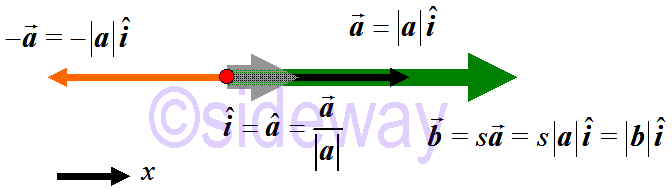Consider a one-dimensional vector 𝒂, a unit vector 𝒊 can always be obtained such that vector 𝒂 can be expressed in terms of the unit vector by multiplying a scalar quantity, the magnetude of the vector, that is 𝒂=|𝒂|𝒊. Therefore the scalar multiplication of a one-dimensional vector can be expressed as the multiplication of a scalar 𝑠 to the magnetude |𝒂| of a vector 𝒂 with respect to the unit vector 𝒂 or 𝒊 of the vector. That is 𝑠𝒂=𝑠|𝒂|𝒊. And the negation of a vector always reverse the vector in opposite direction.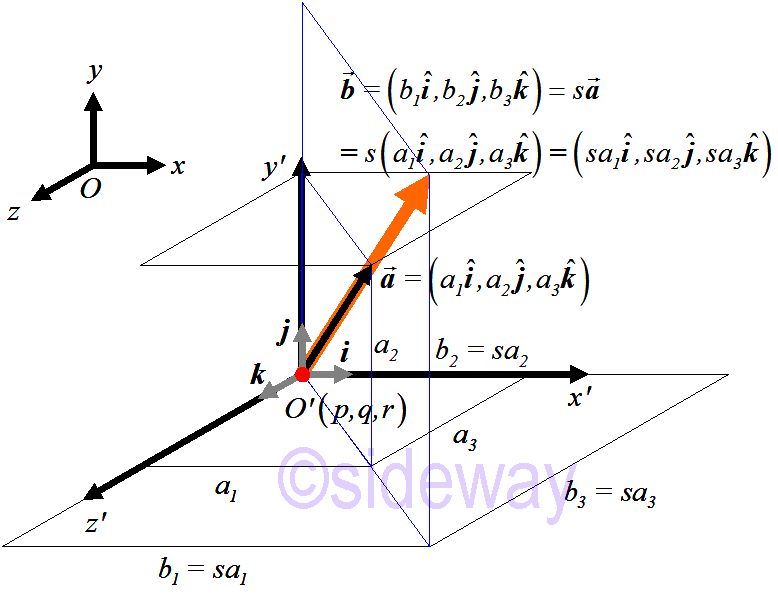For a vector in space, the vector can be decomposited into components with respect to the rectangular Cartesian coordinate system. Each component of the vetor can be considered as one individual dimension. By the triangular approach, the scalar multiplication of a vector in space can also be expressed as the multiplication of a scalar to the magnetude of each component with respect to the unit vector accordingly. That is 𝑠𝒂=𝑠(𝑎1,𝑎2,𝑎3)=(𝑠𝑎1,𝑠𝑎2,𝑠𝑎3)

#### Scalar Multiplication Rule

```Scalar Multiplication of a VectorLet 𝒂=(𝑎1,𝑎2,𝑎3) and 𝑠 be any real number 𝒃=𝑠𝒂=𝑠(𝑎1,𝑎2,𝑎3)=(𝑠𝑎1,𝑠𝑎2,𝑠𝑎3)=(𝑏1,𝑏2,𝑏3)```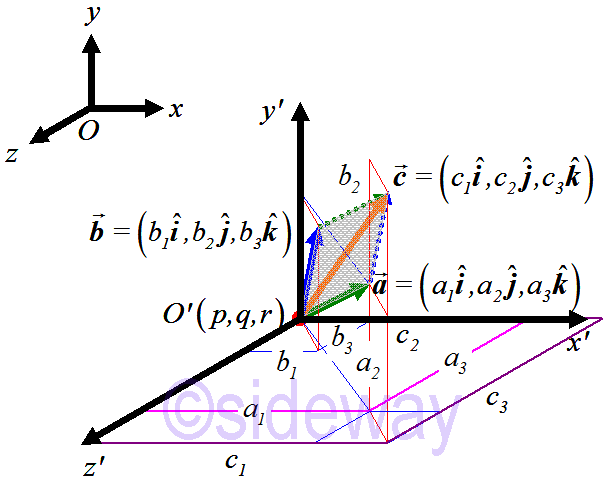Geometrically, addition of two coinitial vectors, i.e. 𝒂+𝒃, can be visualized by using the parallelogram law through putting the initial point of second vector 𝒃 at the terminal point of first vector 𝒂 because two coinitial vectors are always coplanar vectors. The resultant vector 𝒄 can be obtained by joining the initial point of first vector to the terminal point of second vector. That is 𝒄=𝒂+𝒃=(𝑎1,𝑎2,𝑎3)+(𝑏1,𝑏2,𝑏3)=(𝑎1+𝑏1,𝑎2+𝑏2,𝑎3+𝑏3)=(𝑐1,𝑐2,𝑐3)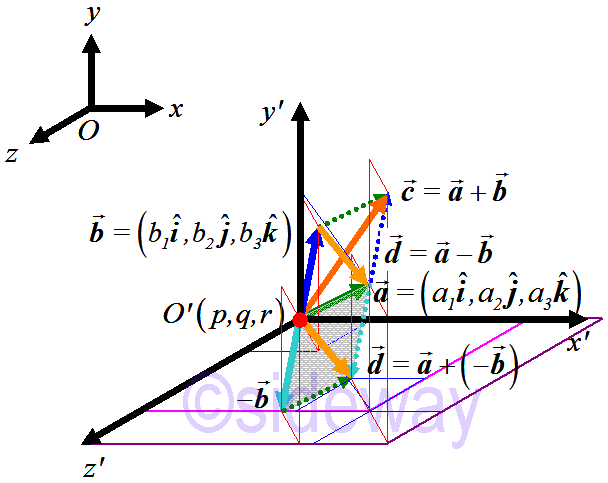Similarly, subtraction of two coinitial vectors, i.e. 𝒂𝒃, can also be visualized by using the parallelogram law through putting the initial point of the negation of second vector 𝒃, i.e. −𝒃, at the terminal point of first vector 𝒂. The resultant vector 𝒅 can be obtained by joining the initial point of first vector to the terminal point of negation of second vector as in addition of vectors. That is 𝒅=𝒂+(−𝒃)
Besides, the subtraction of two coinitial vectors can be constructed directly from vectors 𝒂 and 𝒃 by the basic definition of vector, that is 𝒅=𝒂𝒃=𝐵𝐴=−𝐴𝐵=−(𝒃𝒂).

```Addition of VectorsLet 𝒂=(𝑎1,𝑎2,𝑎3) and 𝒃=(𝑏1,𝑏2,𝑏3) be coinitial vectors 𝒄=𝒂+𝒃=(𝑎1,𝑎2,𝑎3)+(𝑏1,𝑏2,𝑏3)=(𝑎1+𝑏1,𝑎2+𝑏2,𝑎3+𝑏3)=(𝑐1,𝑐2,𝑐3) ```

## Vector Product Operation of Vectors

Vector product operation of vectors are rules developed for specific product operation between two vectors. In general, the product of two vectors can be expressed as following. ```Let 𝒂=(𝑎1𝒊,𝑎2𝒋,𝑎3𝒌), 𝒃=(𝑏1𝒊,𝑏2𝒋,𝑏3𝒌) 𝒂𝒃=𝑎1𝒊𝑏1𝒊+𝑎1𝒊𝑏2𝒋+𝑎1𝒊𝑏3𝒌+𝑎2𝒋𝑏1𝒊+𝑎2𝒋𝑏2𝒋+𝑎2𝒋𝑏3𝒌+𝑎3𝒌𝑏1𝒊+𝑎3𝒌𝑏2𝒋+𝑎3𝒌𝑏3𝒌 𝒂𝒃=𝑎1𝑏1𝒊𝒊+𝑎2𝑏2𝒋𝒋+𝑎3𝑏3𝒌𝒌+𝑎1𝑏2𝒊𝒋+𝑎1𝑏3𝒊𝒌+𝑎2𝑏1𝒋𝒊+𝑎2𝑏3𝒋𝒌+𝑎3𝑏1𝒌𝒊+𝑎3𝑏2𝒌𝒋 𝒂𝒃=(𝑎1𝑏1𝒊𝒊+𝑎2𝑏2𝒋𝒋+𝑎3𝑏3𝒌𝒌)+(𝑎1𝑏2𝒊𝒋+𝑎2𝑏1𝒋𝒊+𝑎1𝑏3𝒊𝒌+𝑎3𝑏1𝒌𝒊+𝑎2𝑏3𝒋𝒌+𝑎3𝑏2𝒌𝒋) ```

### Dot Product of Two Vectors

Dot product is commonly called scalar product because dot product of two vectors gives a scalar value along either vector. Dot product is also called inner product because dot product of two vectors is the sum of products of corresponding components of two vectors. The dot product operation of two vectors is developed to determine the along effect of one vector's component that along with another vector.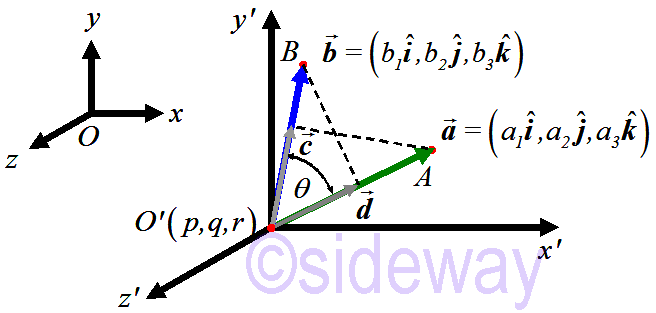Therefore the dot product of two coinitial vectors, 𝒂 and 𝒃 can be interpreted as the magnitude of one vector multiplies by the perpendicular projection of another vector on the previous one taken with the appropriate sign. In other words, the dot product between two vectors gives a scalar result with no direction.

According to the definition of dot product, the dot product of any unit vector dotted with itself is equal to 1, and the dot product magnetude of any two different unit vectors is equal to 0.

```𝒊∙𝒊=𝒋∙𝒋=𝒌∙𝒌=𝟏∙𝟏=cos(0)𝟏=𝟏, undetermined, along either vector 𝒊∙𝒋=𝒌∙𝒊=𝒋∙𝒌=𝟏∙𝟏=cos(90)𝟏=𝟎 𝒋∙𝒊=𝒊∙𝒌=𝒌∙𝒋=𝟏∙𝟏=cos(−90)𝟏=𝟎 Let 𝒂=(𝑎1𝒊,𝑎2𝒋,𝑎3𝒌), 𝒃=(𝑏1𝒊,𝑏2𝒋,𝑏3𝒌) 𝒂∙𝒃=(𝑎1𝑏1𝒊∙𝒊+𝑎2𝑏2𝒋∙𝒋+𝑎3𝑏3𝒌∙𝒌)+(𝑎1𝑏2𝒊∙𝒋+𝑎2𝑏1𝒋∙𝒊+𝑎1𝑏3𝒊∙𝒌+𝑎3𝑏1𝒌∙𝒊+𝑎2𝑏3𝒋∙𝒌+𝑎3𝑏2𝒌∙𝒋) ⇒𝒂∙𝒃=𝑎1𝑏1𝒊∙𝒊+𝑎2𝑏2𝒋∙𝒋+𝑎3𝑏3𝒌∙𝒌 ⇒𝒂∙𝒃=𝑎1𝑏1+𝑎2𝑏2+𝑎3𝑏3, undetermined along either vector ``` The dot product can be visualized geometrically in two-dimensional space.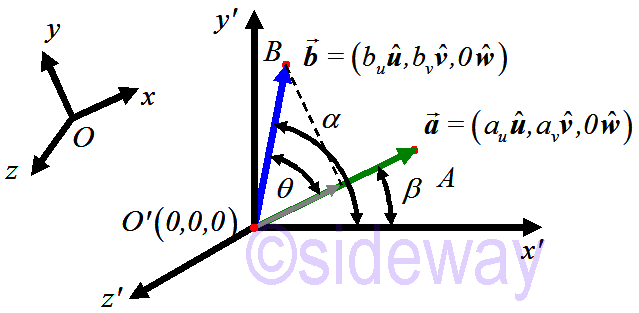Select another rectangular Cartesian coordinate system such that vectors 𝒂 and 𝒃 lie on the 𝑥𝑦-plane. The angles difference identity for cosine can then be used to derived the dot product of two vectors. And can further be extended to three-dimentional space. ```Let 𝒂=(𝑎𝑢𝒖,𝑎𝒗𝒗,𝑎𝑤𝒘)=(𝑎𝑢𝒖,𝑎𝒗𝒗,0𝒘), 𝒃=(𝑏𝑢𝒖,𝑏𝑣𝒗,𝑏𝑤𝒘)=(𝑏𝑢𝒖,𝑏𝑣𝒗,0𝒘) 𝒂=|𝒂|𝒂, 𝒃=|𝒃|𝒃, 𝒂∙𝒃=|𝒃|cos 𝜃 𝒂, 𝒃∙𝒂=|𝒂|cos 𝜃 𝒃 𝒂∙𝒃=|𝒂|𝒂∙𝒃=|𝒂||𝒃|cos 𝜃 𝒂 𝒃∙𝒂=|𝒃|𝒃∙𝒂=|𝒃||𝒂|cos−𝜃 𝒃=|𝒃||𝒂|cos 𝜃 𝒃 ⇒undetermined mathematically, either along 𝒂 or 𝒃 in direction ⇒𝒂∙𝒃=𝒃∙𝒂=|𝒂||𝒃|cos 𝜃=|𝒂||𝒃|(cos 𝛼 cos 𝛽+sin 𝛼 sin 𝛽)=|𝒂||𝒃|𝑏𝑢|𝒃|𝑎𝑢|𝒂|+𝑏𝒗|𝒃|𝑎𝒗|𝒃| ⇒𝒂∙𝒃=𝑎𝑢𝑏𝑢+𝑎𝑣𝑏𝑣 (direction in either 𝒂 or 𝒃)``` Value of dot product can be verified trigonometrically by applying the law of cosine to the triangle ⧍𝑂𝐴𝐵 with 𝜃 is the angle between vectors 𝒂 and 𝒃. ```Let 𝑂𝐴=𝒂=(𝑎1,𝑎2,𝑎3), 𝑂𝐵=𝒃=(𝑏1,𝑏2,𝑏3) ⇒|𝐴𝐵|2=(𝑏1−𝑎1)2+(𝑏2−𝑎2)2+(𝑏3−𝑎3)2=|𝐵𝐴|2=(𝑎1−𝑏1)2+(𝑎2−𝑏2)2+(𝑎3−𝑏3)2 ⇒|𝐴𝐵|2=|𝒂|2+|𝒃|2−2|𝒂||𝒃|cos 𝜃; law of cosine ⇒(𝑏1−𝑎1)2+(𝑏2−𝑎2)2+(𝑏3−𝑎3)2=(𝑎21+𝑎22+𝑎23)+(𝑎21+𝑎22+𝑎23)−2|𝒂||𝒃|cos 𝜃 ⇒−2𝑎1𝑏1−2𝑎2𝑏2−2𝑎3𝑏3=−2|𝒂||𝒃|cos 𝜃 ⇒|𝒂||𝒃|cos 𝜃=𝑎1𝑏1+𝑎2𝑏2+𝑎3𝑏3 ⇒𝒂∙𝒃=|𝒂||𝒃|cos 𝜃=𝑎1𝑏1+𝑎2𝑏2+𝑎3𝑏3 ``` Therefore angle between two coinitial vectors can be determined by dot product ```|𝒂||𝒃|cos 𝜃=𝑎1𝑏1+𝑎2𝑏2+𝑎3𝑏3 ⇒cos 𝜃=𝑎1𝑏1+𝑎2𝑏2+𝑎3𝑏3|𝒂||𝒃| ⇒𝜃=cos−1𝑎1𝑏1+𝑎2𝑏2+𝑎3𝑏3|𝒂||𝒃| if 𝑎1𝑏1+𝑎2𝑏2+𝑎3𝑏3=0 then 𝜃=90 ```

#### Dot Product Rule

```Dot Product RuleLet 𝒂=(𝑎1,𝑎2,𝑎3) and 𝒃=(𝑏1,𝑏2,𝑏3) be coinitial vectors 𝒂∙𝒃=|𝒂||𝒃|cos 𝜃=𝑎1𝑏1+𝑎2𝑏2+𝑎3𝑏3```

### Cross Product of Two Vectors

Unlike determining the effect of one vector's component that along with another vector by the dot product opereation of two vector, the cross product operation of two vectors is developed to determine the cross effect of one vector's component that perpendicular to another vector. Therefore the axis of cross effect due to the cross product always normal to the plane of the two vectors lying in.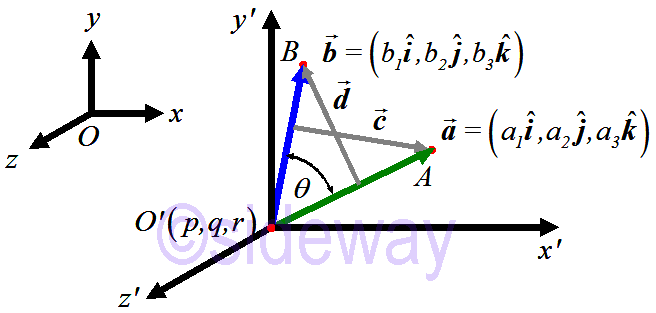According to the definition of cross product, the cross product of any unit vector crossed with itself is equal to 𝟎. The cross product of any two different unit vectors that follows the right hand rule is equal to 𝟏normal, while the cross product of any two different unit vectors that does not follows the right hand rule is equal to −𝟏normal. ```𝒊×𝒊=𝒋×𝒋=𝒌×𝒌=𝟏×𝟏=sin(0)𝟏normal=𝟎 𝒊×𝒋=𝒋×𝒌=𝒌×𝒊=𝟏×𝟏=sin(90)𝟏normal=𝟏normal ⇒𝒊×𝒋=𝒌, 𝒋×𝒌=𝒊, 𝒌×𝒊=𝒋 𝒋×𝒊=𝒌×𝒋=𝒊×𝒌=𝟏×𝟏=sin(−90)𝟏normal=−𝟏normal=−(𝒊×𝒋)=−(𝒋×𝒌)=−(𝒌×𝒊) ⇒𝒋×𝒊=−𝒌, 𝒌×𝒋=−𝒊, 𝒊×𝒌=−𝒋 Let 𝒂=(𝑎1𝒊,𝑎2𝒋,𝑎3𝒌), 𝒃=(𝑏1𝒊,𝑏2𝒋,𝑏3𝒌) 𝒂×𝒃=(𝑎1𝑏1𝒊×𝒊+𝑎2𝑏2𝒋×𝒋+𝑎3𝑏3𝒌×𝒌)+(𝑎1𝑏2𝒊×𝒋+𝑎2𝑏1𝒋∙𝒊+𝑎1𝑏3𝒊×𝒌+𝑎3𝑏1𝒌×𝒊+𝑎2𝑏3𝒋×𝒌+𝑎3𝑏2𝒌×𝒋) ⇒𝒂×𝒃=(𝑎1𝑏1𝒊×𝒊+𝑎2𝑏2𝒋×𝒋+𝑎3𝑏3𝒌×𝒌)+((𝑎1𝑏2−𝑎2𝑏1)𝒊×𝒋+(𝑎2𝑏3−𝑎3𝑏2)𝒋×𝒌+(𝑎3𝑏1−𝑎1𝑏3)𝒌×𝒊) ⇒𝒂×𝒃=(𝑎2𝑏3−𝑎3𝑏2)𝒊+(𝑎3𝑏1−𝑎1𝑏3)𝒋+(𝑎1𝑏2−𝑎2𝑏1)𝒌 ⇒𝒂×𝒃=𝒊 𝒋 𝒌𝑎1 𝑎2 𝑎3𝑏1 𝑏2 𝑏3𝒊 𝒋𝑎1 𝑎2𝑏1 𝑏2 , determinant form: ↘ multiplication gives +value; ↙ multiplication gives −value ``` Magnetude of cross product can be considered geometrically as the area of a parallelogram with 𝜃 is the angle between vectors 𝒂 and 𝒃.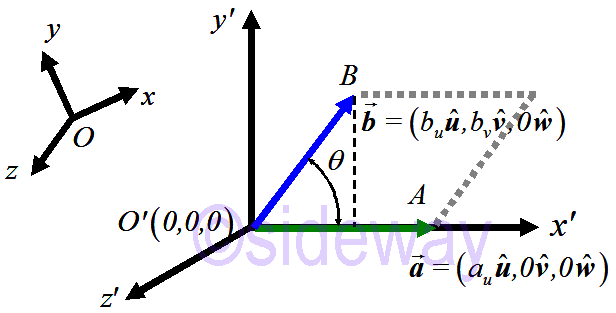Select another rectangular Cartesian coordinate system such that vectors 𝒂 and 𝒃 lie on the 𝑥𝑦-plane and vector 𝒂 lies on the 𝑥-axis. ```Let 𝒂=(𝑎𝑢𝒖,𝑎𝒗𝒗,𝑎𝑤𝒘)=(𝑎𝑢𝒖,0𝒗,0𝒘), 𝒃=(𝑏𝑢𝒖,𝑏𝑣𝒗,𝑏𝑤𝒘)=(𝑏𝑢𝒖,𝑏𝑣𝒗,0𝒘) 𝒂×𝒃=(𝑎𝒗𝑏𝑤−𝑎𝑤𝑏𝒗)𝒖+(𝑎𝑤𝑏𝑢−𝑎𝑢𝑏𝑤)𝒗+(𝑎𝑢𝑏𝒗−𝑎𝒗𝑏𝑢)𝒘 ⇒|𝒂×𝒃|2=(𝒂×𝒃)∙(𝒂×𝒃)=(𝑎𝒗𝑏𝑤−𝑎𝑤𝑏𝒗)2+(𝑎𝑤𝑏𝑢−𝑎𝑢𝑏𝑤)2+𝑎𝑢𝑏𝒗−𝑎𝒗𝑏𝑢)2 ⇒|𝒂×𝒃|2=(𝑎𝒗𝑏𝑤)2−2(𝑎𝒗𝑏𝑤𝑎𝑤𝑏𝒗)+(𝑎𝑤𝑏𝒗)2+(𝑎𝑤𝑏𝑢)2−2(𝑎𝑤𝑏𝑢𝑎𝑢𝑏𝑤)+(𝑎𝑢𝑏𝑤)2 +(𝑎𝑢𝑏𝒗)2−2(𝑎𝑢𝑏𝒗𝑎𝒗𝑏𝑢)+(𝑎𝒗𝑏𝑢)2 ⇒|𝒂×𝒃|2=(𝑎𝑢𝑏𝒗)2, substitute values ⇒|𝒂×𝒃|=𝑎𝑢𝑏𝒗 ∵|𝒂|=𝑎𝑢, |𝒃|sin 𝜃=𝑏𝑣 ⇒|𝒂×𝒃|=|𝒂||𝒃|sin 𝜃=area of parallelogram ``` Therefore angle between two coinitial vectors can be determined by cross product. ```|𝒂||𝒃|sin 𝜃=((𝑎2𝑏3−𝑎3𝑏2)2+(𝑎3𝑏1−𝑎1𝑏3)2+(𝑎1𝑏2−𝑎2𝑏1)2) ⇒sin 𝜃=((𝑎2𝑏3−𝑎3𝑏2)2+(𝑎3𝑏1−𝑎1𝑏3)2+(𝑎1𝑏2−𝑎2𝑏1)2)|𝒂||𝒃| ⇒𝜃=sin−1((𝑎2𝑏3−𝑎3𝑏2)2+(𝑎3𝑏1−𝑎1𝑏3)2+(𝑎1𝑏2−𝑎2𝑏1)2)|𝒂||𝒃| if (𝑎2𝑏3−𝑎3𝑏2)=(𝑎3𝑏1−𝑎1𝑏3)=(𝑎1𝑏2−𝑎2𝑏1)=0, then 𝜃=0 or 𝜃=180 ```

#### Cross Product Rule

```Cross Product RuleLet 𝒂=(𝑎1,𝑎2,𝑎3) and 𝒃=(𝑏1,𝑏2,𝑏3) be coinitial vectors 𝒂×𝒃=|𝒂||𝒃|sin 𝜃=(𝑎2𝑏3−𝑎3𝑏2)𝒊+(𝑎3𝑏1−𝑎1𝑏3)𝒋+(𝑎1𝑏2−𝑎2𝑏1)𝒌```

ID: 130800175 Last Updated: 11/12/2019 Revision: 1 Ref:References

1. Robert C. Wrede, 2013, Introduction to Vector and Tensor Analysis
2. Daniel Fleisch, 2012, A Student’s Guide to Vectors and Tensors
3. Howard Anton, Chris Rorres, 2010, Elementary Linear Algebra: Applications VersionHome 5

Management

HBR 3

Information

Recreation

Culture

Chinese 1097

English 337

Computer

Hardware 149

Software

Application 198

Digitization 117

Numeric 19

Programming

Web 283

Unicode 494

HTML 65

CSS 58

ASP.NET 92

OS 389

Python 19

Knowledge

Mathematics

Algebra 25

Geometry 18

Calculus 67

Engineering

Mechanical

Rigid Bodies

Statics 92

Dynamics 37

Control

Physics

Electric 27